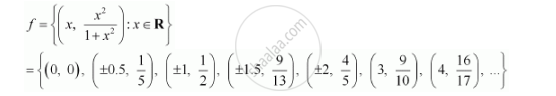CBSE (Arts) Class 11CBSE
Share

# Let F = {(X, X^2/(1+X^2)):X Element R} Be a Function from R into R. Determine the Range of F. - CBSE (Arts) Class 11 - Mathematics

ConceptSome Functions and Their Graphs

#### Question

Let f = {(x, x^2/(1+x^2)):x ∈ R} be a function from R into R. Determine the range of f.

#### SolutionThe range of f is the set of all second elements. It can be observed that all these elements are greater than or equal to 0 but less than 1.

[Denominator is greater numerator]

Thus, range of f = [0, 1)

Is there an error in this question or solution?

#### APPEARS IN

NCERT Solution for Mathematics Textbook for Class 11 (2018 to Current)
Chapter 2: Relations and Functions
Ex. 2.40 | Q: 6 | Page no. 46

#### Video TutorialsVIEW ALL 

Solution Let F = {(X, X^2/(1+X^2)):X Element R} Be a Function from R into R. Determine the Range of F. Concept: Some Functions and Their Graphs.
S